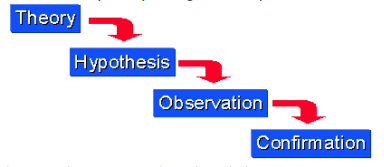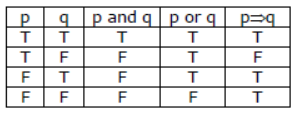# CBSE Class 11 Mathematics Mathematical Reasoning Notes

Download CBSE Class 11 Mathematics Mathematical Reasoning Notes in PDF format. All Revision notes for Class 11 Mathematics have been designed as per the latest syllabus and updated chapters given in your textbook for Mathematics in Class 11. Our teachers have designed these concept notes for the benefit of Class 11 students. You should use these chapter wise notes for revision on daily basis. These study notes can also be used for learning each chapter and its important and difficult topics or revision just before your exams to help you get better scores in upcoming examinations, You can also use Printable notes for Class 11 Mathematics for faster revision of difficult topics and get higher rank. After reading these notes also refer to MCQ questions for Class 11 Mathematics given on studiestoday

## Revision Notes for Class 11 Mathematics Chapter 14 Mathematical Reasoning

Class 11 Mathematics students should refer to the following concepts and notes for Chapter 14 Mathematical Reasoning in Class 11. These exam notes for Class 11 Mathematics will be very useful for upcoming class tests and examinations and help you to score good marks

### Chapter 14 Mathematical Reasoning Notes Class 11 Mathematics

Class XI

Chapter:14 Mathematical Reasoning

Chapter Notes

Key Concepts

1. There are two types of reasoning the deductive and inductive. Deductive reasoning was developed by Aristotle, Thales, Pythagoras in the classical Period (600 to 300 B.C.).

2. In deduction, given a statement to be proven, often called a conjecture or a theorem, valid deductive steps are derived and a proof may or may not be established. Deduction is the application of a general case to a particular case.

3. Inductive reasoning depends on working with each case, and developing a conjecture by observing incidence till each and every case is observed.

4. Deductive approach is known as the top-down" approach”. Given the theorem which is narrowed down to specific hypotheses then to observation. Finally the hypotheses is tested with specific data to get the confirmation (or not) of original theory.5. Mathematical reasoning is based on deductive reasoning. The classic example of deductive reasoning, given by Aristotle, is
· All men are mortal.
· Socrates is a man.
· Socrates is mortal.6. The basic unit involved in reasoning is mathematical statement.

7. A sentence is called a mathematically acceptable statement if it is either true or false but not both. A sentence which is both true and false simultaneously is called a paradox.

8. Sentences which involve tomorrow, yesterday, here, there etc i.e variables etc are not statements.

9. The sentence expresses a request, a command or is simply a question are not statements.

10.The denial of a statement is called the negation of the statement.

11.Two or more statements joined by words like “and” “or” are called Compound statements. Each statement is called a component statement. “and” “or” are connecting words.

12.An “ And” statement is true if each of the component statement is true and it is false even if one component statement is false.

13.An “OR” statement is will be true when even one of its components is true and is false only when all its components are false

14.The word “OR” can be used in two ways (i) Inclusive OR (ii) Exclusive OR. If only one of the two options is possible then the OR used is Exclusive OR. If any one of the two options or both the options are possible then the OR used is Inclusive OR.

15.There exists “\$” and “For all” " are called quantifiers.

16.A statement with quantifier “There exists” is true, if it is true for at least one case.

17.If p and q are two statements then a statement of the form 'If p then q' is known as a conditional statement. In symbolic form p implies q is denoted by p Þ q.

18.The conditional statement p Þ q can be expressed in the various other forms:

(i) q if p (ii) p only if q (iii) p is sufficient for q (iv) q is necessary for p.

19. A statement formed by the combination of two statements of the form if p then q and if q then p is p if and only if q. It is called conditional statement.

20. Contrapositive and converse can be obtained by a if then statement
The contrapositive of a statement p ⇒ q is the statement - q ⇒ -p
The converse of a statement p ⇒ q is the statement q ⇒ p

21. Truth values of various statement22. Two prove the truth of an if p- then q statement . there are two ways : the first is assume p is true and prove q is true. This is called the direct method.
Or assume that q is false and prove p is false. This is called the Contrapositive method.

23. To prove the truth of “ p if and only if q” statement , we must prove two things , one that the truth of p implies the truth of q and the second that the truth of q implies the truth of p.

24. The following methods are used to check the validity of statements:
(i) Direct method
(ii) Contra positive method
(iv) Using a counter example
25. To check whether a statement p is true , we assume that it is not true, i.e. ∼p is true . Then we arrive at some result which contradicts our assumption.

 CBSE Class 11 Mathematics Set Theory Notes
 CBSE Class 11 Mathematics Relations And Functions Notes
 CBSE Class 11 Mathematics Complex Numbers Quadratic Equations Notes
 CBSE Class 11 Mathematics Linear Inequalities Notes
 CBSE Class 11 Mathematics Permutation And Combination Formulas And Questions CBSE Class 11 Mathematics Permutation And Combination Notes
 CBSE Class 11 Mathematics Sequence And Series Formulas And Questions CBSE Class 11 Mathematics Sequence And Series Notes
 CBSE Class 11 Mathematics Straight Lines Formulas And Questions
 CBSE Class 11 Mathematics Coordinate Geometry Formulas And Questions CBSE Class 11 Mathematics Three Dimensional Geometry Notes
 CBSE Class 11 Mathematics Limits And Derivatives Notes
 CBSE Class 11 Mathematics Mathematical Reasoning Notes
 CBSE Class 11 Mathematics Statistics Notes
 CBSE Class 11 Mathematics Probability Notes

## More Study Material

### CBSE Class 11 Mathematics Chapter 14 Mathematical Reasoning Notes

We hope you liked the above notes for topic Chapter 14 Mathematical Reasoning which has been designed as per the latest syllabus for Class 11 Mathematics released by CBSE. Students of Class 11 should download and practice the above notes for Class 11 Mathematics regularly. All revision notes have been designed for Mathematics by referring to the most important topics which the students should learn to get better marks in examinations. Studiestoday is the best website for Class 11 students to download all latest study material.

### Notes for Mathematics CBSE Class 11 Chapter 14 Mathematical Reasoning

Our team of expert teachers have referred to the NCERT book for Class 11 Mathematics to design the Mathematics Class 11 notes. If you read the concepts and revision notes for one chapter daily, students will get higher marks in Class 11 exams this year. Daily revision of Mathematics course notes and related study material will help you to have a better understanding of all concepts and also clear all your doubts. You can download all Revision notes for Class 11 Mathematics also from www.studiestoday.com absolutely free of cost in Pdf format. After reading the notes which have been developed as per the latest books also refer to the NCERT solutions for Class 11 Mathematics provided by our teachers

#### Chapter 14 Mathematical Reasoning Notes for Mathematics CBSE Class 11

All revision class notes given above for Class 11 Mathematics have been developed as per the latest curriculum and books issued for the current academic year. The students of Class 11 can rest assured that the best teachers have designed the notes of Mathematics so that you are able to revise the entire syllabus if you download and read them carefully. We have also provided a lot of MCQ questions for Class 11 Mathematics in the notes so that you can learn the concepts and also solve questions relating to the topics. All study material for Class 11 Mathematics students have been given on studiestoday.

#### Chapter 14 Mathematical Reasoning CBSE Class 11 Mathematics Notes

Regular notes reading helps to build a more comprehensive understanding of Chapter 14 Mathematical Reasoning concepts. notes play a crucial role in understanding Chapter 14 Mathematical Reasoning in CBSE Class 11. Students can download all the notes, worksheets, assignments, and practice papers of the same chapter in Class 11 Mathematics in Pdf format. You can print them or read them online on your computer or mobile.

#### Notes for CBSE Mathematics Class 11 Chapter 14 Mathematical Reasoning

CBSE Class 11 Mathematics latest books have been used for writing the above notes. If you have exams then you should revise all concepts relating to Chapter 14 Mathematical Reasoning by taking out a print and keeping them with you. We have also provided a lot of Worksheets for Class 11 Mathematics which you can use to further make yourself stronger in Mathematics

Where can I download latest CBSE Class 11 Mathematics Chapter 14 Mathematical Reasoning notes

You can download notes for Class 11 Mathematics Chapter 14 Mathematical Reasoning for latest academic session from StudiesToday.com

Can I download the Notes for Chapter 14 Mathematical Reasoning Class 11 Mathematics in Pdf format

Yes, you can click on the link above and download notes PDFs for Class 11 Mathematics Chapter 14 Mathematical Reasoning which you can use for daily revision

Are the revision notes available for Chapter 14 Mathematical Reasoning Class 11 Mathematics for the latest CBSE academic session

Yes, the notes issued for Class 11 Mathematics Chapter 14 Mathematical Reasoning have been made available here for latest CBSE session

How can I download the Chapter 14 Mathematical Reasoning Class 11 Mathematics Notes pdf

You can easily access the link above and download the Class 11 Notes for Mathematics Chapter 14 Mathematical Reasoning for each topic in Pdf

Is there any charge for the Class 11 Mathematics Chapter 14 Mathematical Reasoning notes

There is no charge for the notes for CBSE Class 11 Mathematics Chapter 14 Mathematical Reasoning, you can download everything free of charge

Which is the best online platform to find notes for Chapter 14 Mathematical Reasoning Class 11 Mathematics

www.studiestoday.com is the best website from which you can download latest notes for Chapter 14 Mathematical Reasoning Mathematics Class 11

Where can I find topic-wise notes for Class 11 Mathematics Chapter 14 Mathematical Reasoning

Come to StudiesToday.com to get best quality topic wise notes for Class 11 Mathematics Chapter 14 Mathematical Reasoning

Can I get latest Chapter 14 Mathematical Reasoning Class 11 Mathematics revision notes as per CBSE syllabus

We have provided all notes for each topic of Class 11 Mathematics Chapter 14 Mathematical Reasoning as per latest CBSE syllabus